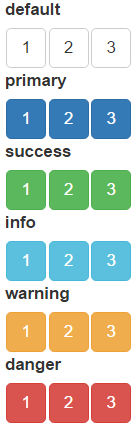# The orderInput

#### 2020-02-03

The orderInput display a list of strings and return their order as a vector in shiny input value. Users can drag and drop the strings to change their display order, and the binding shiny input value will update accordingly. Here is a simple example:

server <- function(input, output) {
output$order <- renderPrint({input$foo_order})
}

ui <- fluidPage(
orderInput(inputId = 'foo', label = 'A simple example', items = c('A', 'B', 'C')),
verbatimTextOutput('order')
)

shinyApp(ui, server)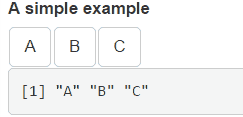As showed in the above example, use input\$inputId_order to get current order, where the “inputId” is the inputId parameter passed to the orderInput function.

The items parameter can either accept a list, an atomic vector or a factor. Based on the type of data provided, the displayed strings and their corresponding order (returned in the shiny input value) may not always the same.

• For fully named list and vector (e.g. c(A = "a", B = "b")), orderInput display names (A and B) but return values (a and b) as order.
• For unnamed or partial named list and vector (e.g. c("a", B = "b")), values (a and b) are used both for display and order.
• For factor (e.g. factor("a", "b")), values (a and b) are displayed while it’s levels (1 and 2) are used for order.

Multiple orderInputs can be connected to each other by passing their inputIds to the connect parameter. When connected, items from one orderInput can be dragged to another. See the following example:

# items in A can be dragged to B
orderInput('A', 'A', items = 1:3, connect = 'B')
# items in B can be dragged to A
orderInput('B', 'B', items = 4:6, connect = 'A')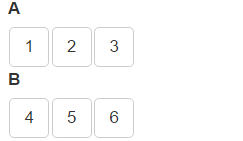A connected orderInput can run in source mode by setting as_source = TRUE. If an item is dragged from a source-mode-orderInput to other orderInputs, the item will not be removed from the original orderInput, that is, drag become copy instead of cut. See the following demonstration:

# In source mode, items dragged to B are copied
orderInput('A', 'A', items = 1:3, connect = 'B', as_source = TRUE)
orderInput('B', 'B', items = 4:6)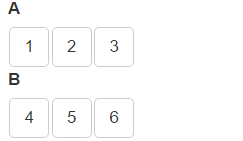If placeholder is set, orderInput shows it when there is no item in it:

orderInput('A', 'A', items = 1:3, connect = 'B')
orderInput('B', 'B', items = NULL, placeholder = 'Drag item here...')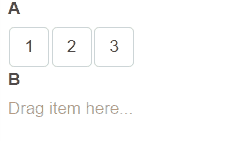orderInput uses the six predefined Bootstrap button classes to style the displayed items. Choose one of them and set in the item_class parameter:

orderInput('default', 'default', items = 1:3, item_class = 'default')
orderInput('primary', 'primary', items = 1:3, item_class = 'primary')
orderInput('success', 'success', items = 1:3, item_class = 'success')
orderInput('info', 'info', items = 1:3, item_class = 'info')
orderInput('warning', 'warning', items = 1:3, item_class = 'warning')
orderInput('danger', 'danger', items = 1:3, item_class = 'danger')